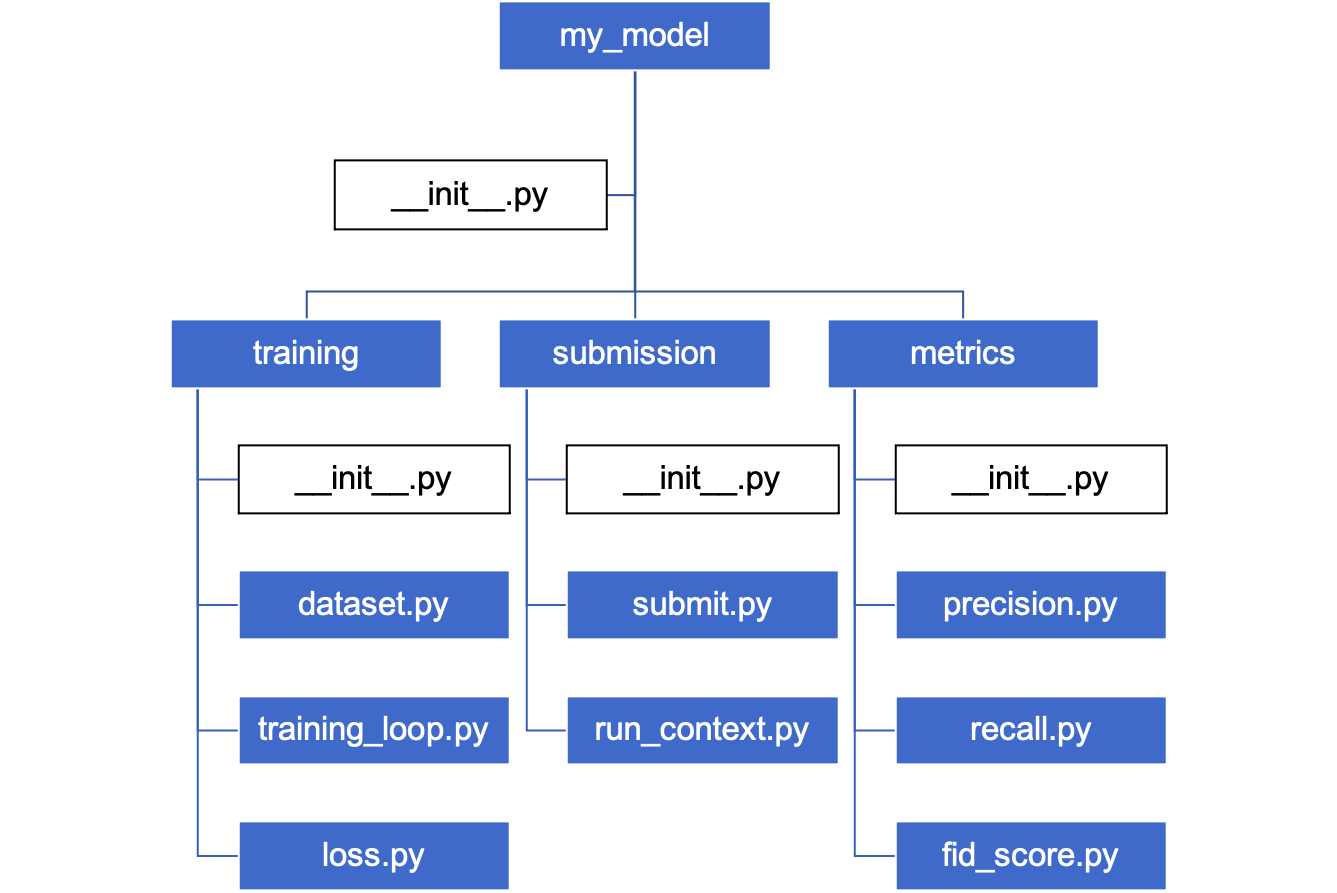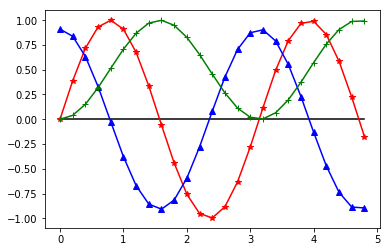ControlNet

## 包和模块

Python可以通过导入(import)关键字使用来自于外部的代码。在Python中，包(package)基本上是可以视为一系列模块(module)组成的目录。而在第五章提到，模块是一个包含了定义和语句的Python源代码文件。Fig. 1. 一个Python的包和模块的例子。Adapted from 

## 库和框架

• 用于文字处理
• string, re, difflib
• 用于数学计算
• math, ramdom, statistics
• 用于函数式编程
• itertools, functools, operator
• 用于文件访问
• os, shutil, pathlib
• 用于文件压缩和解压
• zlib, gzip
• 用于操作系统服务
• os, io, time, subprocess
• 用于并行计算
• multiprocessing, threading, concurrent

## 标准库

### math

math标准库提供了一些常用的数学函数，主要是在不使用更加复杂的第三方库的情况下可以直接使用。

• 求幂和对数
• exp(x), log(x, base), pow(x, y), …
• 三角函数
• sin(x), cos(x), tan(x), …

• pi, e, inf, nan

### random

random标准库提供了创建假随机数(pesudo-random number)的方法。

• 返回一个随机浮点数，它在[0, 1)之间。

• 返回一个随机整数，它在[a, b]之间。

• choice(seq): 返回序列中的一个随机元素。
• shuffle(seq): 打乱序列中的元素。
• sample(seq, k): 从序列中随机获取k个元素。

## 第三方库

Python的第三方库是外部开发者提供的，所以并不会预先和Python一起安装。所以在使用第三方库之前，我们需要先安装它。

### NumPy

NumPy是一个用C编写的数学计算第三方库。很多其他优秀的第三方库都是基于NumPy来实现的，比如说SciPy, pandas。

NumPy的核心是提供了一个新的数据结构: ndarray(多维数组)。

• 1D数组: np.array([1, 2, 3])
• 2D数组: np.array([[1, 2, 3], [4, 5, 6]])

### SciPy

SciPy是一个NumPy的扩展包，提供了很多用于科学计算的算法，其中包括

• 线性代数
• 微积分
• 统计分布
• 优化
• 聚类
• 图像处理
• 信号处理

### Matplotlib

Matplotlib是一个Python的2D/3D绘图库，主要用于数据可视化，其中包括直方图，柱状图，散点图，等等。

• 一些常用的函数：plot(), hist(), scatter(), show()

### pandas

pandas是一个用于结构化数据处理和分析的Python库。

dict创建:Fig. 2. pandas 备忘录。Adapted from 

## 实践试试

### 可视化数学函数